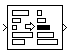# 2-D Median

2-D Median values of input array

• Library:
• Computer Vision Toolbox / Statistics

•## Description

The 2-D Median block computes the median value of an input array. The median value of an array is calculated by using these steps.

1. The values in the matrix are sorted in numeric order.

2. To find the median, of an array, A, with a total number of elements, p, where p is:

• Odd — Median = A(`ceil`(p / 2))

• Even — Median = (A (p / 2) + A (( p / 2) + 1)) / 2

## Ports

### Input

expand all

Specify the Input array as a vector, matrix, or N-D array.

Data Types: `double` | `single` | `int8` | `int16` | `int32` | `uint8` | `uint16` | `uint32` | `fixed point`
Complex Number Support: Yes

### Output

expand all

The median values are returned as scalar, vector, matrix, orN array. The size of the output and how median is calculated depends on the setting of the Find the median value over parameter.

• For M-by-N,the input array, the value of the Find the median value over parameter determines the output form in this manner.

• `Entire input` —The output is the median value of all the elements of theM-by-N input array as a scalar

• `Each row` — The output is a M-by-1 column vector, where each element in the vector is the median value of the corresponding row in the input array.

• `Each column` — The output is a 1-by-N row vector, where each element in the vector is the median value of the corresponding column in the input array.

• `Specified dimension` — If the Dimension parameter is set to `1`, the output is the same when you select `Each column`. If the Dimension parameter is set to `2`, the output is the same as when you select `Each row`. If the Dimension parameter is set to `3`, the output at each sample time is an M-by-N matrix containing the median value of each vector over the third dimension of the input

• For an N-D array, the value of the Find the median value over parameter determines the output form in this manner.

• `Entire input` — The output is the median value of all elements of the M-by-N-by-P input array as scalar.

• `Each row` — The output is a M-by-1-by-P array, where each element contains the median value of each row vector in each plane.

• `Each column` — The output dimension is a 1-by-N-by-P array, where each element contains the median value of each column vector for each plane.

• `Specified dimension` — The output depends on Dimension. If Dimension is set to `1`, the output is the same as when you select `Each column`. If Dimension is set to `2`, the output is the same as when you select `Each row`. If Dimension is set to `3`, the output dimension is a M-by-N matrix containing the median value of each vector over the third dimension of the input

Data Types: `single` | `double` | `int8` | `int16` | `int32` | `uint8` | `uint16` | `uint32` | `fixed point`

## Parameters

expand all

Main

Specify the algorithm by which to sort the input array.

• `Quick sort` — This sorting algorithm uses a recursive sort method and is faster at sorting more than 32 elements.

• `Insertion sort` — This sorting algorithm uses a non-recursive method and is faster at sorting fewer than 32 elements.

When you generate code, to avoid recursive function calls, use the `Insertion sort` algorithm.

Specify the dimension of the input in which the block computes the median.

• `Entire input` — Computes the median over the entire input. The output value is scalar

• `Each row` — Computes the median over each row of the input. The output is column vector

• `Each column` — Computes the median over each column of the input. The output is row vector

• `Specified dimension` — Computes the median over the dimension of the input specified in the Dimension parameter.

• If Dimension is `1`, the output is the same as when you select ```Each column```

• If Dimension is `2`, the output is the same as when you select ```Each row```

Specify the dimension (one-based value) of the input array, over which the block computes the median. The value of this parameter must not exceed the number of dimensions in the input array.

#### Dependencies

To enable this parameter, set the Find the median value over parameter to `Specified dimension`.

Data Types Tab

For details on the fixed-point block parameters, see Specify Fixed-Point Attributes for Blocks.

Select this parameter to prevent the fixed-point tools from overriding the data types you specify in this block. For more information, see Lock the Output Data Type Setting (Fixed-Point Designer).

Note

Floating-point inheritance takes precedence over the data type settings defined on this pane. When inputs are floating point, the block ignores these settings, and all internal data types are floating point.

## Block Characteristics

 Data Types `double` | `fixed point` | `integer` | `single` Multidimensional Signals `no` Variable-Size Signals `yes`

## Extended Capabilities

### Functions

Introduced before R2006a